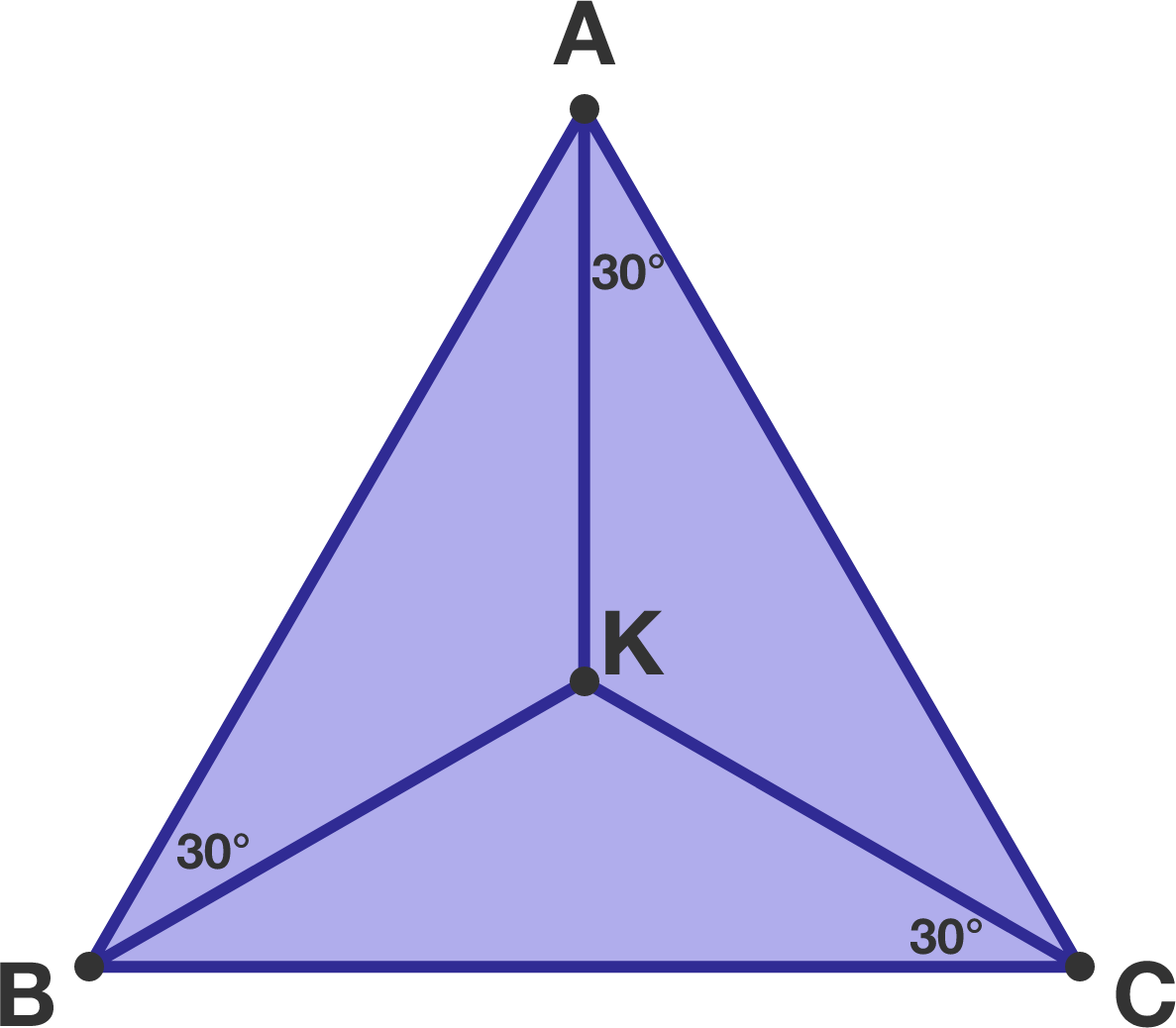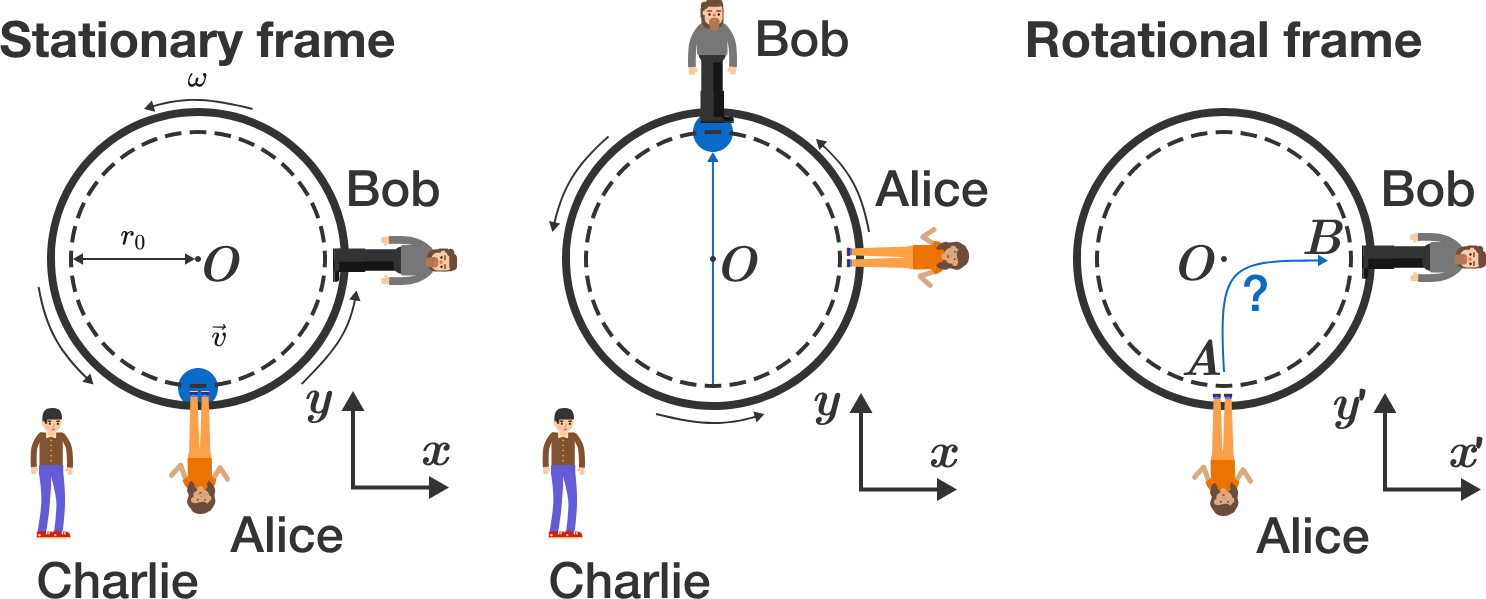# Problems of the Week

Contribute a problemIn triangle $ABC,$ there exists a point $K$ inside of it such that

$\angle{ABK}= \angle{BCK}= \angle{CAK}=30^\circ.$

Is it necessarily true that triangle $ABC$ is equilateral?

The sequence $(-1), {\large (-1)^ \frac{1}{(-1)} }, {\Large (-1) ^ \frac{1}{(-1)^ \frac{1}{(-1)}} }, \ldots$ clearly converges to the integer $-1.$

The sequence $(4), {\large (4)^ \frac{1}{(4)} }, {\Large (4) ^ \frac{1}{(4)^ \frac{1}{(4)}} }, \ldots$ converges to the integer 2. (Can you prove it?)

Does there exist another value $b \neq 1, -1, 4$ such that the sequence $\{a_n\}$ defined recursively by $a_0 = b; \quad a_{n+1} = {\large b^{\frac{1}{a_n}} }$ also converges to an integer?

Note: Written out, this sequence is $(b), {\large (b)^ \frac{1}{(b)} }, {\Large (b) ^ \frac{1}{(b)^ \frac{1}{(b)}} }, \ldots.$

Define a positive integer $n$ to be totatively prime if the set of all positive integers less than $n$ that are relatively prime to $n$ contains no composite numbers. What is the largest totatively prime number?

For example, 9 is not totatively prime because 4 is less than 9 and is relatively prime to 9, but 4 is composite.

Find the largest possible $n$ such that $\big\lfloor \sqrt{1} \big\rfloor + \big\lfloor \sqrt{2} \big\rfloor + \big\lfloor \sqrt{3} \big\rfloor + \cdots + \big\lfloor \sqrt{n} \big\rfloor$is a prime number.

Clarification: $\lfloor x \rfloor$ returns the largest integer less than or equal to $x$.

Alice and Bob are having fun throwing a ball to each other on a merry-go-round. Charlie looks at the game from outside of the merry-go-round. From his perspective, the ball thrown by Alice flies straight along the $y$-axis at a constant velocity of $\vec v = v_0 \vec e_y$ to Bob. Bob can catch this ball after the flight time $t_0 = T/4,$ because the merry-go-round has completed a quarter turn in the meantime.But how does Alice observe (rotating reference system)? What average speed (average of absolute value of velocity vector) $\overline{v'} = \frac{1}{t_0} \int_0^{t_0} |\vec v\,'| dt$ does the ball have from Alice's perspective?

Give the answer in units of $v_0$ and with an accuracy of 3 decimal places.


Hints:

• The merry-go-round rotates with constant frequency $\omega = 2 \pi/T$, so that Alice $($point $r_A)$ and Bob $($point $r_B)$ move on circular paths in the stationary reference system. In the rotating reference system, both points $r_A^\prime$ and $r_B^\prime$ are stationary.
• Search for a $2\times 2$ matrix $\mathbf{D}$ such that the transformation reads $\vec{r}^\prime = \mathbf{D} \cdot \vec{r}$. For the calculation of the average velocity, you may use the integral: $\int \sqrt{1 + x^2} d x = \frac{1}{2} \left( x \sqrt{1 + x^2} + \text{arcsinh}(x) \right).$

Bonus question: Which (fictitious) forces act on the ball in the rotating reference system? How can we explain the path of the ball?

×